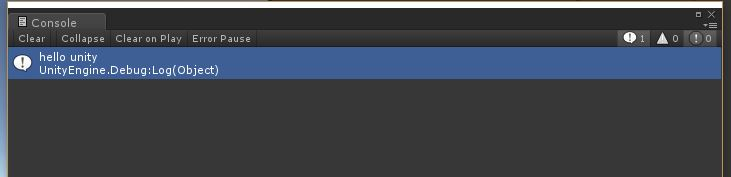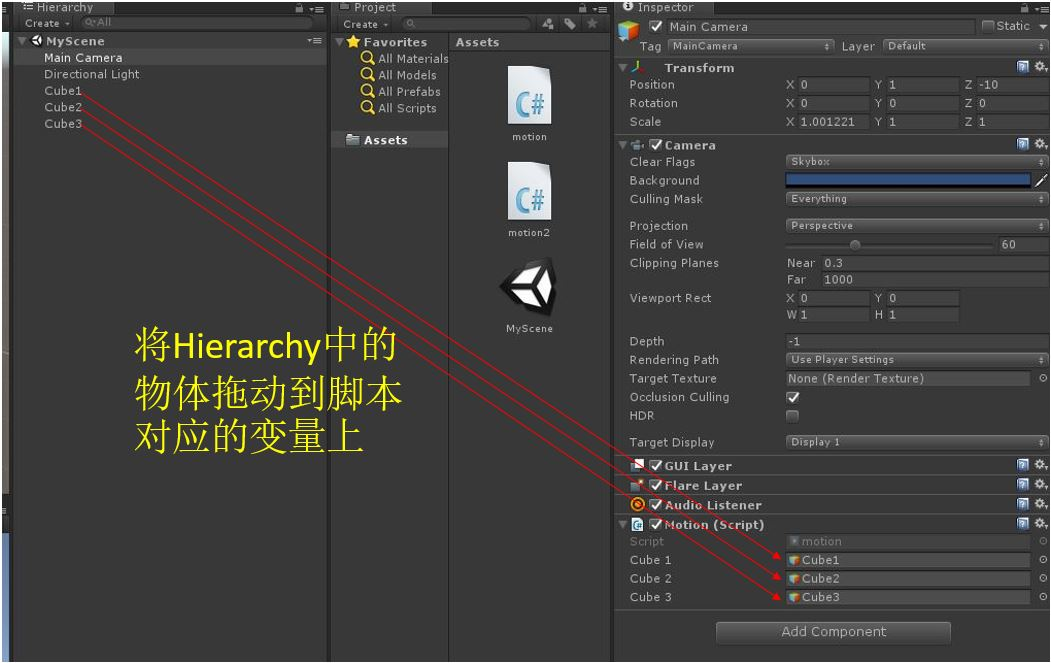# 【Unity3D入门教程】使用C#脚本控制游戏对象

1 创建脚本

using UnityEngine;
using System.Collections;

public class motion : MonoBehaviour {

// Use this for initialization
void Start () {

}

// Update is called once per frame
void Update () {

}
}

2 控制方块运动

Debug.Log("hello unity");（1）旋转

this.transform.Rotate(Vector3.up*1,Space.World);

this.transform.Rotate(Vector3.up*1,Space.Self);

（2）平移

this.transform.Translate(Vector3.up*0.1f,Space.World);

this.transform.Translate(Vector3.up*0.1f,Space.Self);

（3）缩放

this.transform.localScale = new Vector3(1 +Mathf.Sin(Time.time), 1 + Mathf.Sin(Time.time), 1 + Mathf.Sin(Time.time));

3 控制多个对象（1）使用公有变量

using UnityEngine;
using System.Collections;

public class motion : MonoBehaviour {

public GameObject cube1;
public GameObject cube2;
public GameObject cube3;

void Start () {
Debug.Log("hello unity");
}

// Update is called once per frame
void Update () {
cube1.transform.Translate(Vector3.up*0.1f, Space.World);
cube2.transform.Rotate(Vector3.up*1,Space.Self);
cube3.transform.localScale = new Vector3(1 + Mathf.Sin(Time.time), 1 + Mathf.Sin(Time.time), 1 + Mathf.Sin(Time.time));
}
}（2）使用脚本动态绑定

using UnityEngine;
using System.Collections;

public class motion : MonoBehaviour
{

GameObject cube1;
GameObject cube2;
GameObject cube3;

void Start()
{
cube1 = GameObject.Find("Cube1");
cube2 = GameObject.Find("Cube2");
cube3 = GameObject.Find("Cube3");
}

// Update is called once per frame
void Update()
{
cube1.transform.Translate(Vector3.up * 0.1f, Space.World);
cube2.transform.Rotate(Vector3.up * 1, Space.Self);
cube3.transform.localScale = new Vector3(1 + Mathf.Sin(Time.time), 1 + Mathf.Sin(Time.time), 1 + Mathf.Sin(Time.time));
}
}

（3）运行效果如下图10-0503-01
10-262557
07-16160
09-012万+
06-063567
10-162502
08-251122
01-038670
©️2020 CSDN 皮肤主题: Age of Ai 设计师:meimeiellie